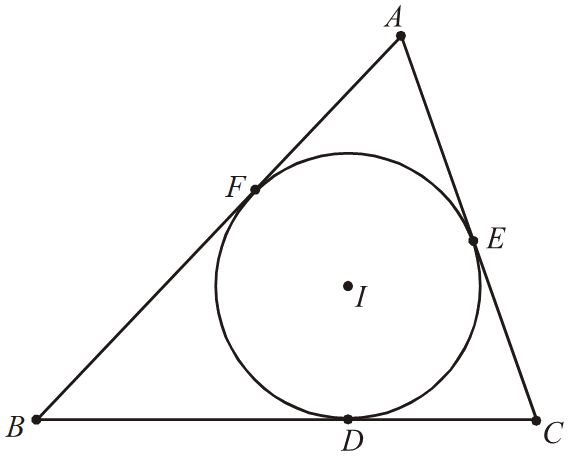# Trigonometry Set-7

Go back to  'SOLVED EXAMPLES'

Example - 17

Let $$ABC$$ be a triangle such that

\begin{align}{\left( {\cot \frac{A}{2}} \right)^2} + {\left( {2\cot \frac{B}{2}} \right)^2} + {\left( {3\cot \frac{C}{2}} \right)^2} = {\left( {\frac{{6s}}{{7r}}} \right)^2}\end{align}

where $$s$$ and $$r$$ denote its semiperimeter and its inradius, respectively. Prove that triangle $$ABC$$ is similar to a triangle $$T$$ whose side lengths are all positive integers with no common divisor and determine these integers.

Soluton: Define

\begin{align}u = \cot \frac{A}{2},\; & v = \cot \frac{B}{2},\; & w = \cot \frac{C}{2}.\end{align}

As shown in Figure, denote the incenter of triangle $$ABC$$ by $$I,$$ and let $$D, E,$$ and $$F$$ be the points of tangency of the incircle with sides $$BC, CA,$$and $$AB,$$ respectively. Then $$\left| EI \right|\text{ }=r$$, and by the standard formula, $$\left| AE \right|\text{ }=s-a.$$We have

\begin{align}u = \cot \frac{A}{2} = \frac{{|AE|}}{{|EI|}} = \frac{{s - a}}{r},\end{align}

and similarly \begin{align}v = \frac{{s - b}}{r},\;w = \frac{{s - c}}{r}\end{align}. Because

\begin{align}\frac{s}{r} = \frac{{(s - a) + (s - b) + (s - c)}}{r} = u + v + w,\end{align}

we can rewrite the given relation as

\begin{align}49\left[ {{u^2} + 4{v^2} + 9{w^2}} \right] = 36{(u + v + w)^2}\end{align}

Expanding the last equality and canceling like terms, we obtain

\begin{align}13{u^2} + 160{v^2} + 405{w^2} - 72(uv + vw + wv) = 0,\end{align}

or

\begin{align}{(3u - 12\upsilon )^2} + {(4\upsilon - 9\omega )^2} + {(18\omega - 2u)^2} = 0\end{align}

Therefore, \begin{align}u:v:w = 1:\frac{1}{4}:\frac{1}{9}\end{align}. This can also be realized by recognizing that the given relation corresponds to equality in Cauchy-Schwarz inequality.

\begin{align}({6^2} + {3^2} + {2^2})\left[ {{u^2} + {{(2v)}^2} + {{(3w)}^2}} \right] \ge {(6 \cdot u + 3 \cdot 2v + 2 \cdot 3w)^2}\end{align}

After multiplying by $$r,$$ we see that

\begin{align} \frac{{s - a}}{{36}} & = \frac{{s - b}}{9} = \frac{{s - c}}{4} = \frac{{2s - b - c}}{{9 + 4}} = \frac{{2s - c - a}}{{4 + 36}} = \frac{{2s - a - b}}{{36 + 9}}\\ & = \frac{a}{{13}} = \frac{b}{{40}} = \frac{c}{{45}}; \end{align}

that is, triangle $$ABC$$ is similar to a triangle with side lengths 13, 40, 45.

Example - 18

Prove that the average of the numbers

\begin{align}2\sin 2{}^\text{o},\ \ 4\sin 4{}^\text{o},\ \ 6\sin 6{}^\text{o},\ ...,\ \ 180\sin 180{}^\text{o}\end{align}

is \begin{align}\cot {{1}^{{}^\circ }}\end{align}.

Solution: We need to prove that

\begin{align}2\sin 2{}^\text{o}+4\sin 4{}^\text{o}+.....+178\sin 178{}^\text{o}=90\cot 1{}^\text{o},\end{align}

which is equivalent to

\begin{align}2\sin 2{}^\text{o}\cdot \sin 1{}^\text{o}+2(2\sin 4{}^\text{o}\cdot \sin 1{}^\text{o})+....+89(2\sin 178{}^\text{o}\cdot \sin 1{}^\text{o})=90\cos 1{}^\text{o}\end{align}

Note that

\begin{align}2\sin 2k{}^\text{o}\sin 1{}^\text{o}=\cos (2k-1){}^\text{o}-\cos (2k+1){}^\text{o}.\end{align}

We have

\begin{align} 2\sin 2{}^\text{o}\cdot &\sin 1{}^\text{o}+2(2\sin 4{}^\text{o}\cdot \sin 1{}^\text{o})+.....+89(2\sin 178{}^\text{o}\cdot \sin 1{}^\text{o}) \\ & =(\cos 1{}^\text{o}-\cos 3{}^\text{o})+2(\cos 3{}^\text{o}-\cos 5{}^\text{o})+.....+89(\cos 177{}^\text{o}-\cos 179{}^\text{o}) \\ & =\cos 1{}^\text{o}+\cos 3{}^\text{o}+....+\cos 177{}^\text{o}-89\cos 179{}^\text{o} \\ & =\cos 1{}^\text{o}+(\cos 3{}^\text{o}+\cos 177{}^\text{o})+.....+(\cos 89{}^\text{o}+\cos 91{}^\text{o})+\ 89\cos 1{}^\text{o} \\ & =\cos 1{}^\text{o}+89\cos 1{}^\text{o}=90\cos 1{}^\text{o}, \end{align} as desired.

Learn from the best math teachers and top your exams

• Live one on one classroom and doubt clearing
• Practice worksheets in and after class for conceptual clarity
• Personalized curriculum to keep up with school